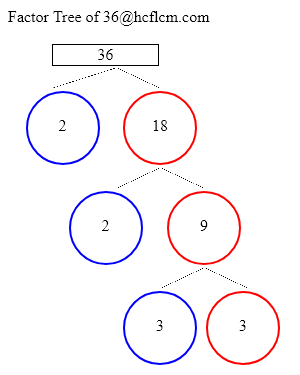# Factors of 36 | Find the Factors of 36 by Factoring Calculator

Factoring Calculator calculates the factors and factor pairs of positive integers.

Factors of 36 can be calculated quickly with the help of Factoring Calculator i.e. 1, 2, 3, 4, 6, 9, 12, 18, 36 positive integers that divide 36 without a remainder.

Factors of 36 are 1, 2, 3, 4, 6, 9, 12, 18, 36. There are 9 integers that are factors of 36. The biggest factor of 36 is 36.

Factors of:

### Factor Tree of 36 to Calculate the Factors

 36 2 18 2 9 3 3Factors of 36 are 1, 2, 3, 4, 6, 9, 12, 18, 36. There are 9 integers that are factors of 36. The biggest factor of 36 is 36.

Positive integers that divides 36 without a remainder are listed below.

• 1
• 2
• 3
• 4
• 6
• 9
• 12
• 18
• 36

• 1 × 36 = 36
• 2 × 18 = 36
• 3 × 12 = 36
• 4 × 9 = 36
• 6 × 6 = 36
• 9 × 4 = 36
• 12 × 3 = 36
• 18 × 2 = 36
• 36 × 1 = 36

### Factors of 36 Table

FactorFactor Number
1one
2two
3three
4four
6six
9nine
12twelve
18eighteen
36thirty six

### How to find Factors of 36?

As we know factors of 36 are all the numbers that can exactly divide the number 36 simply divide 36 by all the numbers up to 36 to see the ones that result in zero remainders. Numbers that divide without remainder are factors and in this case below are the factors

1, 2, 3, 4, 6, 9, 12, 18, 36 are the factors and all of them can exactly divide number 36.

### Frequently Asked Questions on Factors of 36

1. What are the factors of 36?

Answer: Factors of 36 are the numbers that leave a remainder zero. The ones that can divide 36 exactly i.e. factors are 1, 2, 3, 4, 6, 9, 12, 18, 36.

2.What are Factor Pairs of 36?

• 1 × 36 = 36
• 2 × 18 = 36
• 3 × 12 = 36
• 4 × 9 = 36
• 6 × 6 = 36
• 9 × 4 = 36
• 12 × 3 = 36
• 18 × 2 = 36
• 36 × 1 = 36

3. What is meant by Factor Pairs?

Answer:Factor Pairs are numbers that when multiplied together will result in a given product.